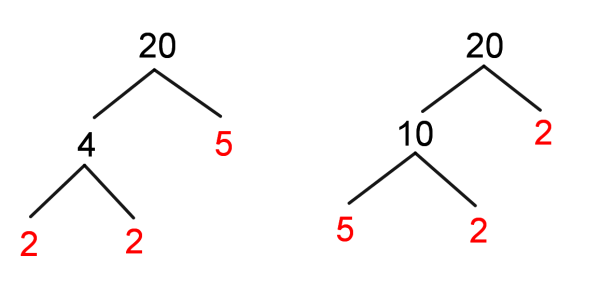# Prime Factorization Calculator

Enter Number
Prime Factors of 10 = 2 x 5
CALCULATE

Prime Factors of a Number calculator finds all prime factors of a positive integer. For small numbers we can calculate the prime factors simply. When it comes to large numbers, finding out the prime factors may be time consuming and bit complicated. To make calculations easy, this calculator will help us to figure out all the prime factors of the given number.
It is necessary to follow the next steps:

1. Enter a number in the box. The number must be positive integer;
2. Press the "CALCULATE" button to make the computation;
3. Prime factors of a number calculator will give all prime factors of the entered number.
Input : A positive integer;
Output : List of prime numbers.

## What is Prime Factors?

The prime factors are the smaller integers found by decomposing the composite number into prime numbers. The product of prime factors forms any positive integer. A prime number is a natural number greater than $1$ that has exactly two factors, $1$ and itself. Negative integers cannot be prime. A composite number is any positive integer that is not prime. Zero and 1 are neither prime nor composite numbers. For example

NumberPrime FactorsPrime/Composite
11/
21,2Prime
31,3Prime
41,2,4Composite
51,5Prime
61,2,3,6Composite
71,7Prime
81,2,4,8Composite
91,3,9Composite
101,2,5,10Composite
111,11Prime
121,2,3,4,6,12Composite
131,13Prime
141,2,7,14Composite
151,3,5,15Composite
161,2,4,8,16Composite
171,17Prime
181,2,3,6,9,18Composite
191,19Prime
201,2,4,5,10,20Composite
211,3,7,21Composite
221,2,11,22Composite
231,23Prime
241,2,3,4,6,8,12,24Composite
251,5,25Composite
261,2,13,26Composite
271,3,9,27Composite
281,2,4,7,14,28Composite
291,29Prime
301,2,3,5,6,10,15,30Composite
311,31Prime
321,2,4,8,16,32Composite
331,3,11,33Composite
341,2,17,34Composite
351,5,7,35Composite
361,2,3,4,6,9,12,18,36Composite
371,37Prime
381,2,19,38Composite
391,3,13,39Composite
401,2,4,5,8,10,20,40Composite
411,41Prime
421,2,3,6,7,14,21,42Composite
431,43Prime
441,2,4,11,22,44Composite
451,3,5,9,15,45Composite
461,2,23,46Composite
471,47Prime
481,2,3,4,6,8,12,16,24,48Composite
491,7,49Composite
501,2,5,10,25,50Composite
511,3,17,51Composite
521,2,4,13,26,52Composite
531,53Prime
541,2,3,6,9,18,27,54Composite
551,5,11,55Composite
561,2,4,7,8,14,28,56Composite
571,3,19,57Composite
581,2,29,58Composite
591,59Prime
601,2,3,4,5,6,10,12,15,20,30,60Composite
611,61Prime
621,2,31,62Composite
631,3,7,9,21,63Composite
641,2,4,8,16,32,64Composite
651,5,13,65Composite
661,2,3,6,11,22,33,66Composite
671,67Prime
681,2,4,17,34,68Composite
691,3,23,69Composite
701,2,5,7,10,14,35,70Composite
711,71Prime
721,2,3,4,6,8,9,12,18,24,36,72Composite
731,73Prime
741,2,37,74Composite
751,3,5,15,25,75Composite
761,2,4,19,38,76Composite
771,7,11,77Composite
781,2,3,6,13,26,39,78Composite
791,79Prime
801,2,4,5,8,10,16,20,40,80Composite
811,3,9,27,81Composite
821,2,41,82Composite
831,83Prime
841,2,3,4,6,7,12,14,21,28,42,84Composite
851,5,17,85Composite
861,2,43,86Composite
871,3,29,87Composite
881,2,4,8,11,22,44,88Composite
891,89Prime
901,2,3,5,6,9,10,15,18,30,45,90Composite
911,7,13,91Composite
921,2,4,23,46,92Composite
931,3,31,93Composite
941,2,47,94Composite
951,5,19,95Composite
961,2,3,4,6,8,12,16,24,32,48,96Composite
971,97Prime
981,2,7,14,49,98Composite
991,3,9,11,33,99Composite
1001,2,4,5,10,20,25,50,100Composite

### How to Find Prime Factorization of a Number?

The prime factors of a number can be found by factor tree. A factor tree is a special diagram for finding the factors of a composite number, until getting all prime factors. For example, let us find the prime factor of 20 by using the factor tree:As we can see, both trees give the same prime factors. There is exactly one prime factorization of 20. The prime factors of 20 is $$20=2\times2\times 5=2^2\times5$$ The fundamental theorem of arithmetic states that every integer greater than $1$ can be factored uniquely into primes (Hardy, G. H., and Wright, E. M., An Introduction to the Theory of Numbers, 5th ed. Oxford, England: Clarendon Press, pp. 3 and 21, 1979.). For instance, $20$ can be factored uniquely into $2\times2\times5$, i.e. there is no other product of primes equal to 20.
Another method for finding the factors of a composite number is called the cake method. To find the prime factors of a composite number, we need to follow the next steps:
• Divide a composite number by its smallest factor;
• Divide a quotient number by its smallest factor;
• Repeat the previous step until the quotient is prime;
• The prime factors are listed.
For example, let us find the the prime factors of 468 by using the cake methodSo, the prime factorization of
$468$ is $468=2\times 2\times3\times 3\times 13=2^2\times 3^2\times 13$.

A monomial can also be factored as a product of prime numbers and variables with no exponent greater than $1$. Negative coefficients can be factored using $-1$ as a factor. For example, the factors of monomials $12xy^2$ are $12xy^2=2\times2\times3\times x\times y\times y$.

### Real World Problems Using Prime Factors

The prime factorization is used in finding the greatest common factor (GFC) and least common multiple (LCM) of two or more numbers. In fraction addition, the LCM is one of the important things. The GCF is useful for simplifying fractions, etc. The prime factorization is of great interest in number theory and application. For instance, the prime factorization is also the essence of Chinese remainder theorem of solving simultaneous congruent equations.

Practice Problem 1:
Find the prime factors of 125.

Practice Problem 2:
Using the prime factorization find the smallest integer number $n$ such that 1960n is a cube number.

The Prime factors of a number calculator, methods, example calculation and practice problems would be very useful for grade school students of K-12 education to learn how to find the prime factorization of a given number. They can use this concept to find the GFC and LCM of a given set of numbers, monomials and polynomials.